Search by Topic

Resources tagged with Working systematically similar to Positive about Negative Numbers:

Filter by: Content type:
Age range:
Challenge level:

There are 321 results

Broad Topics > Using, Applying and Reasoning about Mathematics > Working systematicallyEncouraging Primary Children to Work Systematically

Age 3 to 11

This article for primary teachers suggests ways in which to help children become better at working systematically.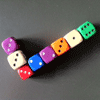I've Submitted a Solution - What Next?

Age 5 to 18

In this article, the NRICH team describe the process of selecting solutions for publication on the site.Maths Trails

Age 7 to 14

The NRICH team are always looking for new ways to engage teachers and pupils in problem solving. Here we explain the thinking behind maths trails.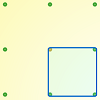Peg and Pin Boards

Age 5 to 11

This article for teachers suggests activities based on pegboards, from pattern generation to finding all possible triangles, for example.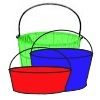More and More Buckets

Age 7 to 11 Challenge Level:

In this challenge, buckets come in five different sizes. If you choose some buckets, can you investigate the different ways in which they can be filled?Consecutive Numbers

Age 7 to 14 Challenge Level:

An investigation involving adding and subtracting sets of consecutive numbers. Lots to find out, lots to explore.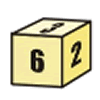First Connect Three for Two

Age 7 to 11 Challenge Level:

First Connect Three game for an adult and child. Use the dice numbers and either addition or subtraction to get three numbers in a straight line.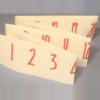Folded Number Line

Age 7 to 11 Challenge Level:

When I fold a 0-20 number line, I end up with 'stacks' of numbers on top of each other. These challenges involve varying the length of the number line and investigating the 'stack totals'.First Connect Three

Age 7 to 14 Challenge Level:

Add or subtract the two numbers on the spinners and try to complete a row of three. Are there some numbers that are good to aim for?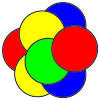Counters in the Middle

Age 7 to 11 Challenge Level:

This task depends on groups working collaboratively, discussing and reasoning to agree a final product.Home Time

Age 7 to 11 Challenge Level:

Alice's mum needs to go to each child's house just once and then back home again. How many different routes are there? Use the information to find out how long each road is on the route she took.Putting Two and Two Together

Age 7 to 11 Challenge Level:

In how many ways can you fit two of these yellow triangles together? Can you predict the number of ways two blue triangles can be fitted together?More on Mazes

Age 7 to 14

There is a long tradition of creating mazes throughout history and across the world. This article gives details of mazes you can visit and those that you can tackle on paper.Nine-pin Triangles

Age 7 to 11 Challenge Level:

How many different triangles can you make on a circular pegboard that has nine pegs?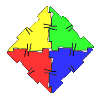Polydron

Age 7 to 11 Challenge Level:

This activity investigates how you might make squares and pentominoes from Polydron.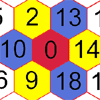Nineteen Hexagons

Age 5 to 7 Challenge Level:

In this maze of hexagons, you start in the centre at 0. The next hexagon must be a multiple of 2 and the next a multiple of 5. What are the possible paths you could take?Cuisenaire Counting

Age 5 to 7 Challenge Level:

Here are some rods that are different colours. How could I make a dark green rod using yellow and white rods?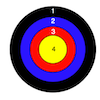Dart Target

Age 7 to 11 Challenge Level:

This task, written for the National Young Mathematicians' Award 2016, invites you to explore the different combinations of scores that you might get on these dart boards.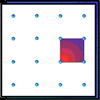Geoboards

Age 7 to 11 Challenge Level:

This practical challenge invites you to investigate the different squares you can make on a square geoboard or pegboard.Arrangements

Age 7 to 11 Challenge Level:

Is it possible to place 2 counters on the 3 by 3 grid so that there is an even number of counters in every row and every column? How about if you have 3 counters or 4 counters or....?An Introduction to Magic Squares

Age 7 to 16

Find out about Magic Squares in this article written for students. Why are they magic?!Finding Fifteen

Age 7 to 11 Challenge Level:

Tim had nine cards each with a different number from 1 to 9 on it. How could he have put them into three piles so that the total in each pile was 15?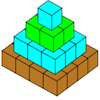Open Squares

Age 7 to 11 Challenge Level:

This task, written for the National Young Mathematicians' Award 2016, focuses on 'open squares'. What would the next five open squares look like?Combining Cuisenaire

Age 7 to 11 Challenge Level:

Can you find all the different ways of lining up these Cuisenaire rods?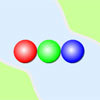Three Ball Line Up

Age 5 to 7 Challenge Level:

Use the interactivity to help get a feel for this problem and to find out all the possible ways the balls could land.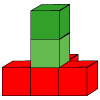Cubes Here and There

Age 7 to 11 Challenge Level:

How many shapes can you build from three red and two green cubes? Can you use what you've found out to predict the number for four red and two green?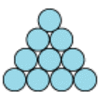Difference

Age 7 to 11 Challenge Level:

Place the numbers 1 to 10 in the circles so that each number is the difference between the two numbers just below it.Find the Difference

Age 5 to 7 Challenge Level:

Place the numbers 1 to 6 in the circles so that each number is the difference between the two numbers just below it.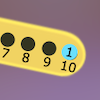Are You Well Balanced?

Age 5 to 7 Challenge Level:

Can you work out how to balance this equaliser? You can put more than one weight on a hook.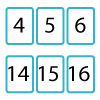Twenty Divided Into Six

Age 7 to 11 Challenge Level:

Katie had a pack of 20 cards numbered from 1 to 20. She arranged the cards into 6 unequal piles where each pile added to the same total. What was the total and how could this be done?Magic Vs

Age 7 to 11 Challenge Level:

Can you put the numbers 1-5 in the V shape so that both 'arms' have the same total?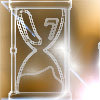Two Egg Timers

Age 7 to 11 Challenge Level:

You have two egg timers. One takes 4 minutes exactly to empty and the other takes 7 minutes. What times in whole minutes can you measure and how?Tiling

Age 7 to 11 Challenge Level:

An investigation that gives you the opportunity to make and justify predictions.Same Length Trains

Age 5 to 7 Challenge Level:

How many trains can you make which are the same length as Matt's, using rods that are identical?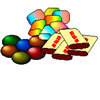The Puzzling Sweet Shop

Age 7 to 11 Challenge Level:

There were chews for 2p, mini eggs for 3p, Chocko bars for 5p and lollypops for 7p in the sweet shop. What could each of the children buy with their money?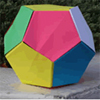Dodecamagic

Age 7 to 11 Challenge Level:

Here you see the front and back views of a dodecahedron. Each vertex has been numbered so that the numbers around each pentagonal face add up to 65. Can you find all the missing numbers?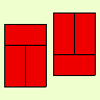Fault-free Rectangles

Age 7 to 11 Challenge Level:

Find out what a "fault-free" rectangle is and try to make some of your own.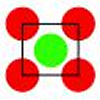Centred Squares

Age 7 to 11 Challenge Level:

This challenge, written for the Young Mathematicians' Award, invites you to explore 'centred squares'.Dice Stairs

Age 7 to 11 Challenge Level:

Can you make dice stairs using the rules stated? How do you know you have all the possible stairs?Being Resourceful - Primary Number

Age 5 to 11 Challenge Level:

Number problems at primary level that require careful consideration.Making Trains

Age 5 to 7 Challenge Level:

Can you make a train the same length as Laura's but using three differently coloured rods? Is there only one way of doing it?Shaping Up

Age 7 to 11 Challenge Level:

Are all the possible combinations of two shapes included in this set of 27 cards? How do you know?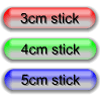Triangle Edges

Age 5 to 7 Challenge Level:

How many triangles can you make using sticks that are 3cm, 4cm and 5cm long?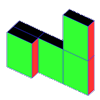Cereal Packets

Age 7 to 11 Challenge Level:

How can you put five cereal packets together to make different shapes if you must put them face-to-face?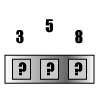Briefcase Lock

Age 5 to 7 Challenge Level:

My briefcase has a three-number combination lock, but I have forgotten the combination. I remember that there's a 3, a 5 and an 8. How many possible combinations are there to try?Factor Lines

Age 7 to 14 Challenge Level:

Arrange the four number cards on the grid, according to the rules, to make a diagonal, vertical or horizontal line.All the Digits

Age 7 to 11 Challenge Level:

This multiplication uses each of the digits 0 - 9 once and once only. Using the information given, can you replace the stars in the calculation with figures?A Mixed-up Clock

Age 7 to 11 Challenge Level:

There is a clock-face where the numbers have become all mixed up. Can you find out where all the numbers have got to from these ten statements?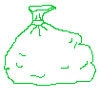Money Bags

Age 5 to 11 Challenge Level:

Ram divided 15 pennies among four small bags. He could then pay any sum of money from 1p to 15p without opening any bag. How many pennies did Ram put in each bag?Multiples Grid

Age 7 to 11 Challenge Level:

What do the numbers shaded in blue on this hundred square have in common? What do you notice about the pink numbers? How about the shaded numbers in the other squares?